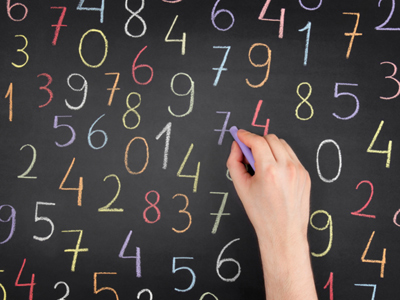You'll have to put lots of numbers into the correct order in this quiz.

# Compare and Order Numbers 3 (Easy)

Comparing and ordering numbers is a fancy way of saying “knowing how much numbers are worth (their value) and putting them in order from largest to smallest (or vice versa)”. If you can count then you should find these questions pretty easy.

This is the third of our Easy level Eleven Plus maths quizzes on the topic. If you haven’t yet played the previous two then I heartily suggest you do. You’ll come across many hints and tips which will help you in your maths lessons at school, in real life situations, and in your exams.

Take your time when playing the quiz. You’d be surprised how often people choose the wrong answer because they didn’t read the question properly. It’s good practise to read a question twice before you pick your answer. Good luck!

1.
How would you arrange the following numbers in ascending order: 15, -9, 22, 0, -17?
22, 15, 0, -9, -17
-17, -9, 0, 15, 22
-17, -9, 22, 15, 0
15, 22, 0, -9, -17
'Ascending order' means that the numbers increase in size as you read from left to right
2.
Which of the following is the biggest number: -1, -11, 0, -111?
-111
-11
-1
0
All the other numbers are negative, so zero is the biggest number. ZERO IS A NUMBER
3.
How many times bigger than 3 is 36?
9 times bigger
8 times bigger
24 times bigger
12 times bigger
36 ÷ 3 = 12, so 36 is 12 times bigger than 3 because there are 12 lots of 3 in 36: 3 + 3 + 3 + 3 + 3 + 3 + 3 + 3 + 3 + 3 + 3 + 3 = 36
4.
What is wrong with this calculation: 14 + 30 - 14 = 44
14 + 30 - 14 = 30
14 + 30 - 14 = -30
14 + 30 - 14 = -14
14 + 30 - 14 = 14
-14 + 14 = 0. Whenever you have two identical numbers that differ only in sign - they cancel each other out to give zero
5.
How would you arrange the following numbers in descending order: 32, 9, -8, -6, 0?
-8, -6, 0, 9, 32
0, -6, -8, 9, 32
32, 9, 0, -6, -8
0, 9, 32, -8, -6,
'Descending order' means that the numbers decrease in size as you read from left to right
6.
Which of the following numbers is the largest: 2, -62, 0, -42?
-62
0
2
-42
2 is the only number greater than zero, so it is the largest
7.
What number lies exactly halfway between 182 and 460?
320
321
322
323
Here's how you solve problems like this: add both numbers together THEN divide by two:
(182 + 460) = 642. So, 642 ÷ 2 = 321
8.
Which number lies exactly between -10 and 90?
30
35
45
40
Here's how you solve problems like this: add both numbers together THEN divide by two:
(-10 + 90) = 80. So, 80 ÷ 2 = 40
9.
Starting from 3, if you move left along the number line 7 places, what number will you be on?
-4
-3
-2
10
Remember that going LEFT means numbers decreasing. If you move LEFT 7 places starting from 3, the numbers become smaller and smaller.
3 - 7 = -4
10.
How many times smaller than 45 is 5?
7 times smaller
8 times smaller
9 times smaller
10 times smaller
45 ÷ 5 = 9, so 45 is 9 times bigger than 5 because there are 9 lots of 5 in 45: 5 + 5 + 5 + 5 + 5 + 5 + 5 + 5 + 5 = 45
Author:  Frank Evans